### Home > MC2 > Chapter 7 > Lesson 7.1.1 > Problem7-11

7-11.
1. A college has a 2:3 ratio of men to women in its student body.
Homework Help ✎1. What is the ratio of women to men?

2. What is the ratio of women to total students?

3. What percent of the college is men?

4. What fraction of the college is women?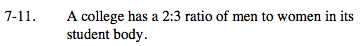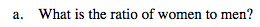If there are 2 men for every 3 women, what would the ratio of women to men be?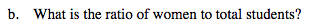If for every 3 women there are 2 men, the total students per 3 women is 5. What is this ratio?

3:5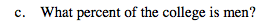If the ratio of men to total students is 2:5, what percentage would this represent?

40%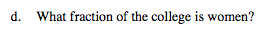Based on the ratio of women to total students from part (b), what would the fraction for women out of total students be?